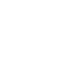# Develop FastHomomorphicApplications Easily

Zama enables web2 and web3
developers to use FHE without
having to learn cryptography.The Concrete Framework is now available. Read more.The Concrete Framework is now available. Read more.The Concrete Framework is now available. Read more.

## Homomorphic encryption enables applications to run privately by processing data blindly.How it Works
01

## Write python code, run it on encrypted data

Zama's Concrete Framework enables data scientists to build models that run on encrypted data, without learning cryptography. Just write Python code and Concrete will convert it to an homomorphic equivalent!

``````
import concrete.numpy as hnp

return x + y

inputset = [(2, 3), (0, 0), (1, 6), (7, 7), (7, 1), (3, 2), (6, 1), (1, 7), (4, 5), (5, 4)]
compiler = hnp.NPFHECompiler(add, {"x": "encrypted", "y": "encrypted"})

print(f"Compiling...")
circuit = compiler.compile_on_inputset(inputset)

examples = [(3, 4), (1, 2), (7, 7), (0, 0)]
for example in examples:
result = circuit.run(*example)
print(f"Evaluation of {' + '.join(map(str, example))} homomorphically = {result}")

``````
02

## Use low-level FHE operators to fine-tune execution

Cryptographers looking to manipulate FHE operators directly can do so using Concrete’s low-level library. Built in Rust using a highly modual architecture, it makes extending Concrete safe and easy.

``````
use concrete::*;

fn main() -> Result<(), CryptoAPIError> {

// generate a secret key
let secret_key = LWESecretKey::new(&LWE128_630);

// the two values to add
let m1 = 8.2;
let m2 = 5.6;

// Encode in [0, 10[ with 8 bits of precision and 1 bit of padding
let encoder = Encoder::new(0., 10., 8, 1)?;

// encrypt plaintexts
let mut c1 = LWE::encode_encrypt(&secret_key, m1, &encoder)?;
let c2 = LWE::encode_encrypt(&secret_key, m2, &encoder)?;

// add the two ciphertexts homomorphically, and store in c1

// decrypt and decode the result
let m3 = c1.decrypt_decode(&secret_key)?;

// print the result and compare to non-FHE addition
println!("Real: {}, FHE: {}", m1 + m2, m3);

Ok(())
}
``````
01

## Concrete ML Makes Use Cases Easy

With Concrete ML, we are able to show some very appealing examples of how the tool can be used with models that are already familiar to data scientists.

``````
from sklearn.datasets import make_classification
from sklearn.model_selection import train_test_split
from concrete.ml.sklearn import LogisticRegression

# Create a synthetic dataset
N_EXAMPLE_TOTAL = 100
N_TEST = 20
x, y = make_classification(n_samples=N_EXAMPLE_TOTAL, class_sep=2, n_features=4, random_state=42)
X_train, X_test, y_train, y_test = train_test_split(
x, y, test_size=N_TEST / N_EXAMPLE_TOTAL, random_state=42)

# Fix the quantization to 3 bits
model = LogisticRegression(n_bits=3)

# Fit the model
model.fit(X_train, y_train)

# We run prediction on non-encrypted data as a reference
y_pred_clear = model.predict(X_test, execute_in_fhe=False)

# We compile into an FHE model
model.compile(x)

# We then run the inference in FHE
y_pred_fhe = model.predict(X_test, execute_in_fhe=True)
print("In clear  :", y_pred_clear)
print("In FHE    :", y_pred_fhe)
print("Comparison:", (y_pred_fhe == y_pred_clear))

``````
03

## Write python code, run it on encrypted data

Data scientists looking to run their models on encrypted data can now do so without learning cryptography. Just build your model using Numpy. Concrete will convert it to an optimized FHE executable!

``````<script>

var tricksWord = document.getElementsByClassName("tricks");
for (var i = 0; i < tricksWord.length; i++) {

var wordWrap = tricksWord.item(i);
wordWrap.innerHTML = wordWrap.innerHTML.replace(/(^|<\/?[^>]+>|\s)([^\s<]+)/g,
'\$1\$2');

}

var tricksLetter = document.getElementsByClassName("tricksword");
for (var i = 0; i < tricksLetter.length; i++) {

var letterWrap = tricksLetter.item(i);
letterWrap.innerHTML = letterWrap.textContent.replace(/\S/g, "\$&");

}

</script>``````